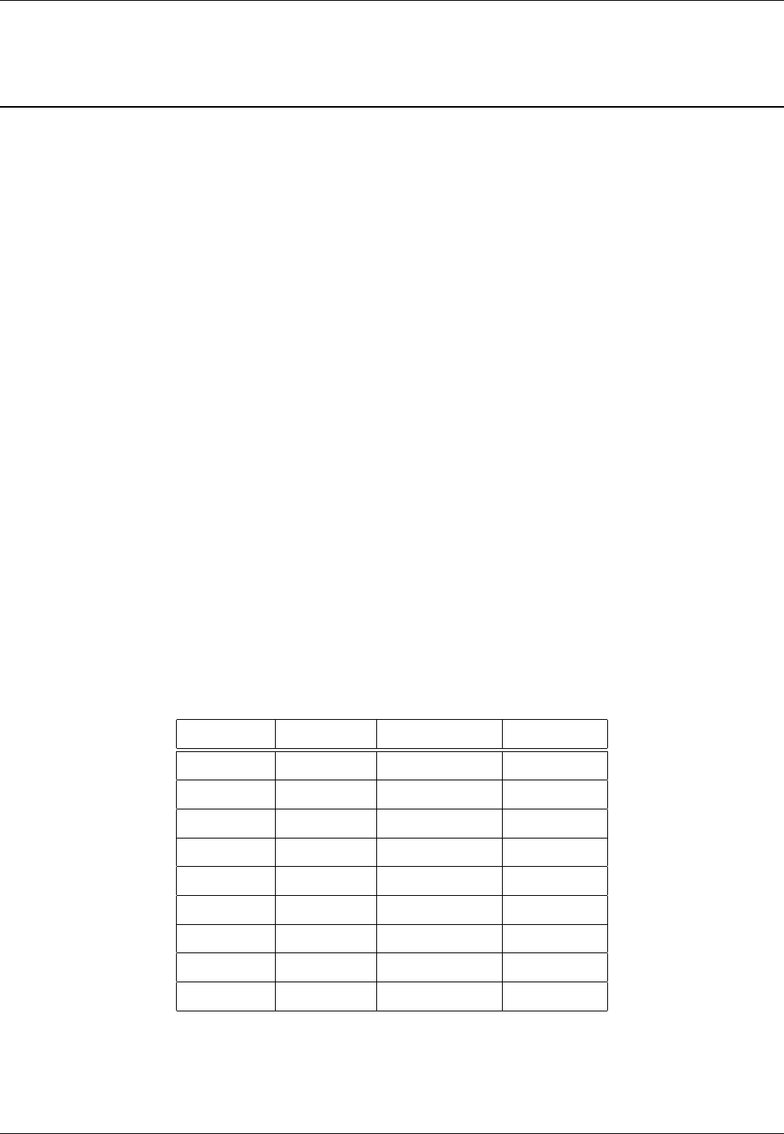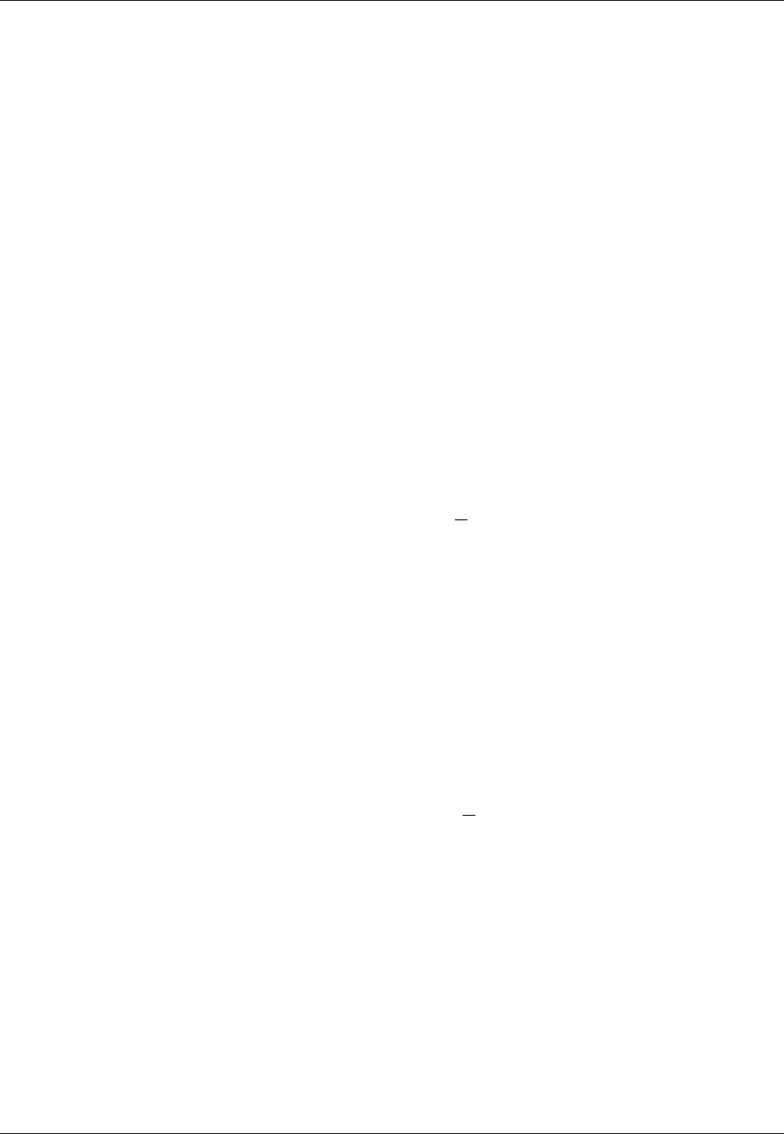# Economics 0011A/B Lecture Notes - Lecture 8: Mira-Bhayandar Municipal Corporation, Gift Card, Problem Set

18 views7 pages
School
Department
ProfessorECO 101H1: Principles of Microeconomics Prof. Freitas, University of Toronto
Problem Set 1: Thinking like an Economist
Solutions
1a) Solution: \$20. Opportunity cost is the value of the next best alternative. If you don’t order the beef you
would get the next preferred option, the chicken which gives you a beneﬁt of \$20. Both dishes cost \$15 and
you use the same gift card to buy it. The resource you are using to get the beef is the same resource you are
using to get your next best alternative which is the chicken. When you choose the beef you are giving up the
enjoyment from chicken which you value at \$20.
1b) Solution: \$25. If you don’t choose the vegetarian you next best alternative is the beef which you value at \$25.
1c) Solution: \$23. You next best option now is the ﬁsh which you value at \$23. Since the vegetarian option, even
with the higher value is not your second most preferred option, it is still not the next best option and hence
does not aﬀect your opportunity cost.
1d) Solution: From an opportunity cost perspective, for every option that is not beef, your opportunity cost is \$25,
the value of the beef. On the other hand, the opportunity cost of beef is \$20. Beef has the lowest opportunity
cost. Beef also has the highest beneﬁt, which means on net (Net beneﬁts = Beneﬁts-Opportunity Cost) beef
is the best option for you. You choose beef.
1e) Solution: Yes it matters. If you’re not very hungry today then you could save the gift card for a later date
when you’re hungrier and your value from that option could aﬀect your calculation of opportunity cost. It
could be also higher than your value from the beef dish and then you would choose to use the card later.
1f) Solution: Yes it matters. If you didn’t have a gift card then you could have spent \$15 in cash at another
restaurant or skipped lunch and spent it on something else. If that is a relevant option we need to know how
it is valued before you make you choice.
2a) Solution: 6 hours.
Whenever you see a “how many?” question your ﬁrst instinct should be to calculate marginal costs and
beneﬁts of an extra unit. Here your units are hours.
The marginal cost of an hour spent studying is the money you could have earned babysitting, which is \$20.
For marginal beneﬁt, we want the extra beneﬁt from each additional unit. For any quantity Q, the marginal
beneﬁt of the Qth hour is the total beneﬁt when you have Qhours minus the total beneﬁt from when you
have Q1 hours. Table 1does this calculation for you.
No. of hours Total Beneﬁt Marginal Beneﬁt Marginal cost
1 \$67 \$67 \$20
2 \$126 \$59 \$20
3 \$177 \$51 \$20
4 \$220 \$43 \$20
5 \$255 \$35 \$20
6 \$282 \$27 \$20
7 \$301 \$19 \$20
8 \$312 \$11 \$20
9\$315 \$3 \$20
Table 1: Marginal beneﬁts and costs of an extra hour spent studying.
For the ﬁrst 6 hours, the marginal beneﬁt is higher than the marginal cost. For the seventh hour, your
additional beneﬁt is \$19 but you are giving up \$20 in babysitting wages so you choose to study only 6 hours.
201909 1 of 7Problem Set 1 Solutions
Unlock document

This preview shows pages 1-2 of the document.
Unlock all 7 pages and 3 million more documents.ECO 101H1: Principles of Microeconomics Prof. Freitas, University of Toronto
2b) Solution: Hint: In ECO101, we want you to get the intuition so we will stick with linear equations. This means
that your equations will look something like the following:
Marginal cost will be MC(Q) = c+dQ, with both cand dnon-negative (i.e., either positive or equal to zero).
Marginal Beneﬁt will be MB(Q) = abQ, with apositive and bnon-negative and most likely positive.
Question for you: why is it reasonable to assume that both aand bare non-negative? Think of stories and
We want to ﬁnd an equation of the formMB(Q) = abQ. From Table 1, we see that marginal beneﬁt
decreases by \$8 with each additional unit. This means b= 8. As M B(1) = 67 and marginal beneﬁt decreases
by \$8 with each additional unit, the intercept (i.e., marginal beneﬁt when quantity equals 0) is 67 + 8 = 75.
We thus have MB(Q) = 75 8Q.
2c) What is your marginal beneﬁt from spending 3.5 hours studying over the weekend? Use the equation you
derived in question above.
Solution: \$47. We plug in 3.5 into the MB(Q)equation to ﬁnd that MB(3.5) = 75 83.5 = 47. The marginal
beneﬁt at the instant you study for 2.5 hours is \$47.
2d) Solution: 6.88 hours (6.875 rounded to 2 decimal places becomes 6.88). Because we assume that hours studying
is a continuous variable, you study until marginal beneﬁt is exactly equal to marginal cost.
marginal beneﬁt = marginal cost
75 8Q= 20
55 = 8Q
Q=55
8= 6.875
2e) Solution: I know that at the optimal quantity, Q, your MB(Q) = M C. If you work 7.5 hours then it must
be that at 7.5 hours
marginal beneﬁt = marginal cost
75 8(7.5) = MC
15 = MC
I can check my calculations by checking what you would choose if your MC was indeed \$15.
marginal beneﬁt = marginal cost
75 8Q= 15
60 = 8Q
Q=60
8= 7.5
2f) Solution: When we work with equations you can always safely assume that optimal quantities don’t have to
be integers, even if I don’t specify it in the question.
Same logic as with ﬁnding the equation for marginal costs. Start with the table and ﬁll in marginal costs
which has been done for you in table 2below.
201909 2 of 7Problem Set 1 Solutions
Unlock document

This preview shows pages 1-2 of the document.
Unlock all 7 pages and 3 million more documents.